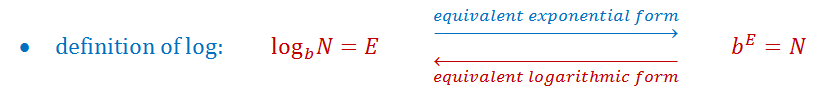# Converting from exponential form to logarithmic form#### Everything You Need in One Place

Homework problems? Exam preparation? Trying to grasp a concept or just brushing up the basics? Our extensive help & practice library have got you covered.#### Learn and Practice With Ease

Our proven video lessons ease you through problems quickly, and you get tonnes of friendly practice on questions that trip students up on tests and finals.#### Instant and Unlimited Help

Our personalized learning platform enables you to instantly find the exact walkthrough to your specific type of question. Activate unlimited help now!

0/0
0/1
##### Examples
###### Lessons
1. Convert from$$exponential form$"$to$$logarithmic form$":$
1. ${2^3} = 8$
2. ${5^0} = 1$
3. ${10^{-2}} = \frac{1}{100}$
4. ${a^{\frac{7}{4}}} = {^4}\sqrt{a^7}$
5. ${e^y} = x$

## Logarithmic form

Logarithms are inverses of exponential functions. It tells us how many times we'll need to multiply a number in order to get another number. For example, if we multiply 2 four times, we'll carry out 2 x 2 x 2 x 2, which gives us 16. When asked how many times we'll need to multiply 2 in order to get 16, the answer is logarithm 4.

## Exponential form

Although this lesson is on the logarithmic form, since logarithms are the inverses of exponential functions, we'll also have to quickly review the exponential form. Exponents is when a number is raised to a certain power that tells you how many times to repeat the multiplication of a number by itself. For example, when you see $2^4$, you'll have to take 2 and multiply it by itself 4 times. That means you'll get a final answer of 16.

## Exponential form to logarithmic form

So how do we switch from the exponential form that we're more familiar with, to the logarithmic form? The conversion is actually pretty simple and is summarized in this definition below:

When you've got an equation in the exponential form of $b^E=N$, how do we write the equation in logarithmic form? In order to convert it to logarithmic form, you'll have to first pay attention to the base. The b is the base in $b^E=N$. This base will be the same as the base in the equation's logarithmic form, and will be denoted by the little $b$ beside log as shown in the definition above. Then, the $E$ and $N$ changes sides so that the $E$ now goes on to the right side of the equation in a logarithm, and the $N$ goes to the left side. And there you have it! By rearranging the components of an exponential form equation around, you'll be able to get to convert to logarithmic form.

## Practice problems

Question 1:

Convert from exponential to logarithmic form:

$2^3=8$

Solution:

We currently have an equation in the form of: $b^E=N$

In order to convert it into the $\log_b N =E$ form, we'll use the definition above. This question's base is 2, so we'll put that beside log as a small 2 on the left side of the new logarithm. Then, we'll switch the 3 and the 8 to the opposite side of where they originally were. This means we'll get the final answer of:

$\log_2 8=3$

Question 2:

Convert exponential to log form:

$10^{-2}=\frac{1}{100}$

Solution:

Once again, we've got an equation in the form of: $b^E=N$

Convert it to this form: $\log_b N =E$. We'll get:

$\log_{10}\frac{1}{100}=-2$

Question 3:

Convert from exponential form to logarithmic form:

$a^{\frac{7}{4}}={^4}\sqrt{a^7}$

Solution:

Convert the equation that we have in its current $b^E=N$ form to $\log_b N =E$ through rearranging the components around. You'll get:

$\log_a {^4}\sqrt{a^7}=\frac{7}{4}$

Next up, you'll learn how to use the richter scale, the ph scale, and the db scale. These are all logarithmic scales and after learning this lesson, you'll understand what logarithms are and the magnitude of the numbers they represent.